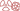THE PHENOMENON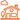IN DAILY LIFE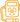QUESTION OF DOSES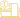AT THE DOCTOR’S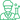At the laboratory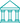At the museum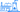NUCLEAR ENERGY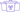A general public knowledge base dedicated to radioactivity created and maintained by the french physics community

# Compton Effect

## Photons as projectiles and electrons as targets

The Compton effect is the name given by physicists to the collision between a photon and an electron. The photon bounces off a target electron and loses energy. These collisions referred as elastic compete with the photoelectric effect when gamma pass through matter. It contributes to their attenuation.

The effect was discovered in 1922 by the american physicist Arthur H. Compton. Compton received the Nobel Prize in Physics in 1927. He demonstrated the particle nature of electromagnetic radiation. It was a sensational discovery at the time.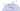Collision between a photon and an atomic electron
The Compton effect occurs with the atomic electrons. A gamma photon plays the role of a projectile that collides with an atomic electron that serves as a target. Gamma is represented as a punctual particle because of its very short wavelength at the atomic scale. As the vast majority of electrons has a smaller energy than the gamma  energy, physicists are accustomed to neglect it and to consider the electron as a target at rest. In the collision, the electron is put in motion at a certain angle, while the gamma scattered loses a part of its energy.

Compton collisions can be viewed as elastic collisions between a photon and an electron. These elastic collisions become predominant when the photon energy becomes large compared to the energy that holds the electron in an atom, its binding energy. For a light atom such as carbon, the Compton effect prevails on the photoelectric effect above 20 keV. For copper it is above 130 keV, and for lead 600 keV.

In this gamma energy range, which is rather extended , the phenomenon concerns all the electrons of the atom, whereas in the photoelectric effect these are the two electrons from the innermost K shell, which play a role. For an absorber, it is the density of electrons that is crucial in the range where Compton effect dominates. Lead has thus also an advantage over lighter materials, even if less important than for the photoelectric effect, which came at the fourth power of the high electrical charge of its nucleus.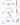Unequal energy sharing
The energy sharing between the gamma photon and the target electron depends on the gamma scattering angle. The most likely case is the one in which the photon is not scattered and do not lose energy. The most rare case is the one where the gamma bounces backward propelling the electron forward. On average, the photon transfers only a small portion of its energy to the electron and undergoes a significant shift in direction (the values in the figure have been calculated for an energy of 500 keV).

The gamma is not destroyed in the collision. The photon that emerges with the electron, called “scattered” photon shares the initial energy with the electron set in motion. The electron then loses its energy by ionization as a beta electron. The scattered gamma propagates through the material without depositing energy until it interacts again.

The energy sharing is unequal. It depends on the angle between the scattered photon and the initial gamma (the gamma probability distribution at a given angle is given by a formula called the “Klein-Nishima formula”). Despite its extremely light mass, the electron is indeed a heavy target for a photon that is massless. The laws of physics governing the Compton effect are such as the scattered photon carries most of the initial energy: 96% on average at 50 keV, 83% at 500 keV.

The scattered photon emerges usually in a different direction than the incident photon one. It can even go backward ( backscattering). On average it scatters with an angle of 30 to 45 degrees. Gamma of hundreds of keV can undergo multiple Compton scattering before being absorbed by photoelectric effect.

When the gamma energy exceeds 1 MeV, which is rarely the case for the gamma rays emitted by nuclei, Compton scattering begins to be challenged by a new phenomenon: the transformation of a gamma into an electron and its antiparticle, a positron. This phenomenon becomes prominent with the high-energy gamma produced by example with particle accelerators.

NEXT: Photoelectric Effect

BremsstrahlungTHE PHENOMENONIN DAILY LIFEQUESTION OF DOSESAT THE DOCTOR’SAt the laboratoryAt the museumNUCLEAR ENERGY